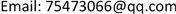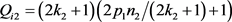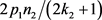﻿ 证明无奇完全数 Proof of No Odd Complete Number

Pure Mathematics
Vol. 09  No. 03 ( 2019 ), Article ID: 30363 , 4 pages
10.12677/PM.2019.93056

Proof of No Odd Complete Number

Shanzhong Zou

Guangzhou GuangdongReceived: Apr. 26th, 2019; accepted: May 6th, 2019; published: May 22nd, 2019ABSTRACT

The odd number is Q, Q = 2N + 1, and N is called odd element of Q. Through the analysis of N, it is proved that there is no odd complete number.

Keywords:Ontology of Odd Numbers, The Ontology of Compound Odd Numbers1. 奇合数体的表示法

$N={P}_{i}n+{S}_{i}$ (1)

2. 命题证明

$N=2{N}_{i1}{N}_{i2}+{N}_{i1}+{N}_{i2}={\sum }_{i=1}^{t}\left({N}_{i1}+{N}_{i2}+1\right)$ (2)

(2)式称为奇完全数的奇体表达式。由(2)可得推论一，N为偶数，t也是偶数。

$\because$ $N\in h$，根据定义1， $N=p{n}_{i}+s$，( $s=\left(p-1\right)/2$,${n}_{i}\ge 1$ )。

$Q={P}_{1}^{{n}_{1}}{p}_{2}^{{n}_{2}}\cdots {p}_{i}^{{n}_{i}}\cdots {p}_{m}^{m}$ 中，设 ${p}_{1}$ 是Q中最小的素数，把 ${N}_{i1}$${N}_{i2}$ 分别表为

${N}_{i1}={p}_{1}{n}_{1}+{k}_{1},{N}_{i2}={p}_{1}{n}_{2}+{k}_{2},\left({n}_{1}\ge 0,{n}_{2}\ge 0,0\le {k}_{1}<{p}_{1},0\le {k}_{2}<{p}_{1}\right)$

${N}_{i1}$${N}_{i2}$ 可以依据组合 $\left\{{p}_{1}{n}_{1}+{k}_{1},{p}_{1}{n}_{2}+{k}_{2}\right\}$ 的不同，形成不同表达式。

$\begin{array}{l}2\left({p}_{1}{n}_{1}+{k}_{1}\right)\left({p}_{1}{n}_{2}+{k}_{2}\right)+{p}_{1}{n}_{1}+{k}_{1}+{p}_{1}{n}_{2}+{k}_{2}\\ =2{p}_{1}^{2}{n}_{1}{n}_{2}+2{p}_{1}{n}_{1}{k}_{2}+2{p}_{1}{n}_{2}{k}_{1}+2{k}_{1}{k}_{2}+{p}_{1}{n}_{1}+{k}_{1}+{p}_{1}{n}_{2}+{k}_{2}\end{array}$

$N={p}_{1}{n}_{i}+{s}_{1}={p}_{1}\left(2{p}_{1}{n}_{1}{n}_{2}+2{n}_{2}{k}_{1}+2{p}_{1}{n}_{1}{k}_{2}+{n}_{1}+{n}_{2}\right)+2{k}_{1}{k}_{2}+{k}_{1}+{k}_{2}$

$N={p}_{1}{n}_{j}+2{k}_{1}{k}_{2}+{k}_{1}+{k}_{2}={\sum }_{i=1}^{t}\left({p}_{1}{n}_{1}+{k}_{1}+{p}_{1}{n}_{2}+{k}_{2}+1\right)$ (3)

${N}_{i1}={p}_{1}{n}_{1}+{k}_{1}$${N}_{i2}={p}_{1}{n}_{2}+{k}_{2}$ 还原为Q，

${Q}_{i1}=2\left({p}_{1}{n}_{1}+{s}_{1}\right)+1={p}_{1}{q}_{1}$,${Q}_{i2}=2\left({p}_{1}{n}_{2}+{k}_{2}\right)+1=2{p}_{1}{n}_{2}+2{k}_{2}+1$,$\because {Q}_{i2}$ 是奇数， $\therefore 2{p}_{1}{n}_{2}/\left(2{k}_{2}+1\right)$ 应该是偶数，要使是偶数，必须满足以下条件：1) ${k}_{2}={s}_{1}$，有 ${p}_{1}/\left(2{k}_{2}+1\right)=1$， 2) ${k}_{2}=0$$2{k}_{2}+1=1$，3) ${n}_{2}=\left(2{k}_{2}+1\right)n$${n}_{2}/\left(2{k}_{2}+1\right)=n$

1) ${k}_{2}={s}_{1}$,

$\because {k}_{1}={s}_{1}$ $\therefore N={p}_{1}{n}_{i}+{s}_{1}=2{p}_{1}{n}_{j}+{p}_{1}{s}_{1}+{s}_{1}={\sum }_{i=1}^{t}\left({p}_{1}{n}_{1}+{p}_{1}{n}_{2}+{s}_{1}+{s}_{1}+1\right)$

$\frac{N}{{p}_{1}}=2{n}_{j}+{s}_{1}+\left({s}_{1}/{p}_{1}\right)={\sum }_{i=1}^{t}\left({n}_{1}+{n}_{2}+1\right)$

$\because {s}_{1}/{p}_{1}$ 不是整数， $\therefore$${k}_{1}={s}_{1}$ 时， ${k}_{2}\ne {s}_{1}$

2) ${k}_{2}=0,{k}_{1}={s}_{1}$

$N={p}_{1}{n}_{i}+{s}_{1}={\sum }_{i=1}^{t}\left({p}_{1}{n}_{1}+{p}_{1}{n}_{2}+{s}_{1}+1\right)=t\left({s}_{1}+1\right)+{\sum }_{i=1}^{t}\left({p}_{1}{n}_{1}+{p}_{1}{n}_{2}\right)$

$\exists !t\left({s}_{1}+1\right)\equiv {s}_{1}\mathrm{mod}{p}_{1}$，即 $t\left({s}_{1}+1\right)={p}_{1}n+{s}_{1}$，使等式成立。

$\because$ t是偶数，设 $t=2{n}_{t}$

$2{n}_{t}{s}_{1}+2{n}_{t}=2{n}_{t}{s}_{1}+{n}_{t}+{n}_{t}={p}_{1}{n}_{t}+{n}_{t}$$\exists !{n}_{t}={p}_{1}n+{s}_{1},n\ge 0$，满足等式，

$\therefore {p}_{1}^{2}n+{p}_{1}{s}_{1}+{p}_{1}n+{s}_{1}={p}_{1}n\left({p}_{1}+1\right)+2{s}_{1}{s}_{1}+{s}_{1}+{s}_{1}$

${N}_{\text{i}1}={p}_{1}{n}_{1}+{s}_{1},{N}_{\text{i}2}={p}_{1}{n}_{2}+{s}_{1}$，这样的结果与 ${k}_{2}={s}_{1}$ 相同， $\therefore {k}_{1}={s}_{1}$${k}_{2}\ne 0$

3) 在 $2{p}_{1}{n}_{2}/\left(2{k}_{2}+1\right)$ 中， ${n}_{2}=\left(2{k}_{2}+1\right)n$

$\because {p}_{1}/\left(2{k}_{2}+1\right)$ 不是整数， $\therefore {n}_{2}/\left(2{k}_{2}+1\right)$ 必须是整数。

$\because$ ${p}_{1}$ 是Q中最小的素数， $\therefore \left(2{k}_{2}+1\right)={p}_{i}$${p}_{i}>{p}_{1}$$\therefore {p}_{1}{n}_{2}+{k}_{2}$ 可以表示为

${p}_{i}{n}_{2}+{s}_{i}$${s}_{i}={k}_{2}$${s}_{i}=\left({p}_{i}-1\right)/2$${p}_{i}$ 是Q中的素数。

$\because 0\le {k}_{2}<{p}_{1}$,$\left(2{k}_{2}+1\right)>{p}_{1}$,$\therefore {k}_{1}<{k}_{2}\le 2{k}_{1}$,

${k}_{1}={m}_{1}$${k}_{2}={m}_{2}$，有 ${k}_{2}-{k}_{1}=\left({m}_{2}-{m}_{1}\right)=\Delta n$，显然 $\Delta n\le {m}_{1}$${k}_{2}=\Delta n+{k}_{1}$

(3)有： $\begin{array}{c}N={p}_{1}{n}_{i}+{s}_{1}\text{}={\sum }_{i=1}^{t}\left({p}_{1}{n}_{1}+{k}_{1}+{p}_{1}{n}_{2}+{k}_{1}+\Delta n+1\right)\\ =t\Delta n+{\sum }_{i=1}^{t}\left({p}_{1}{n}_{1}+{p}_{1}{n}_{2}+{p}_{1}\right)\end{array}$

$\exists !\left(t\Delta n-{s}_{1}\right)/{p}_{1}=n$，即 ${s}_{1}\left(t\Delta n/{s}_{1}-1\right)/{p}_{1}=n$$\because {s}_{1}/{p}_{1}$ 不是整数， $\therefore \left(t\Delta n/{s}_{1}-1\right)/{p}_{1}$ 必须是整数，如果. $\Delta n/{s}_{1}$ 是整数，即 ${k}_{1}={s}_{1},{k}_{2}=2{s}_{1}$$\therefore$ (2)有：

$N=2{p}_{1}{n}_{j}+2×2{s}_{1}{s}_{1}+2{s}_{1}+{s}_{1}={\sum }_{i=1}^{t}\left({p}_{1}{n}_{1}+{p}_{1}{n}_{2}+{s}_{1}+2{s}_{1}+1\right)$

$2{p}_{1}{n}_{j}+{s}_{1}\left(4{s}_{1}+3\right)=t{s}_{1}+{\sum }_{i=1}^{t}\left({p}_{1}{n}_{1}+{p}_{1}{n}_{2}+{p}_{1}\right)$

$\exists !t{s}_{1}={p}_{1}n+{s}_{1}$ 使得 $\left(t{s}_{1}+{\sum }_{i=1}^{t}\left({p}_{1}{n}_{1}+{p}_{1}{n}_{2}+{p}_{1}\right)\right)\equiv {s}_{1}\mathrm{mod}{p}_{1}$

$\left(t{s}_{1}-{s}_{1}\right)/{p}_{1}=n$，即 ${s}_{1}\left(t-1\right)/{p}_{1}=n$$\because {s}_{1}/{p}_{1}$ 不是整数 $\therefore \left(t-1\right)/{p}_{1}=n$，即 $t-1={p}_{1}n$$\because Q={p}_{1}{p}_{2}\cdots {p}_{i}\cdots {p}_{m}$，用Q中 ${p}_{i}$ 逐个代入 $\therefore t-1={p}_{1}{p}_{2}\cdots {p}_{i}\cdots {p}_{m}n$，这使得 ${\sum }_{i=1}^{t}\left({N}_{i1}+{N}_{i2}+1\right)>Q$$\therefore \Delta n\ne {s}_{1}$

$\because \left(t\Delta n/{s}_{1}-1\right)/{p}_{1}=n$$\therefore t\Delta n/{s}_{1}-1={p}_{1}n$$\because \Delta n/{s}_{1}$ 不是整数， $\therefore t/{s}_{1}$ 必须是整数， $\therefore t\Delta n$ 在能整除 ${s}_{1},{s}_{2},\cdots ,{s}_{i},\cdots ,{s}_{m}$ 的同时， $\frac{t\Delta n}{{s}_{1}}-1$ 还必须整除 ${p}_{i}$

$\therefore t>{p}_{1}{p}_{2}\cdots {p}_{i}\cdots {p}_{m}$，这样便造成 ${\sum }_{i=1}^{t}\left({N}_{i1}+{N}_{i2}+1\right)>Q$$\therefore$${k}_{1}={s}_{1}$ 时，因 ${k}_{2}$ 无法取值，原假设不成立，所以不存在奇完全数。证明完！

Proof of No Odd Complete Number[J]. 理论数学, 2019, 09(03): 417-420. https://doi.org/10.12677/PM.2019.93056

1. 1. Gu, C.H. (1992) Mathematics Dictionary. Shanghai Dictionary Press, Shanghai.

2. 2. Min, S.H. (1981) Method of Number Theory. Science Press, Beijing.

NOTES